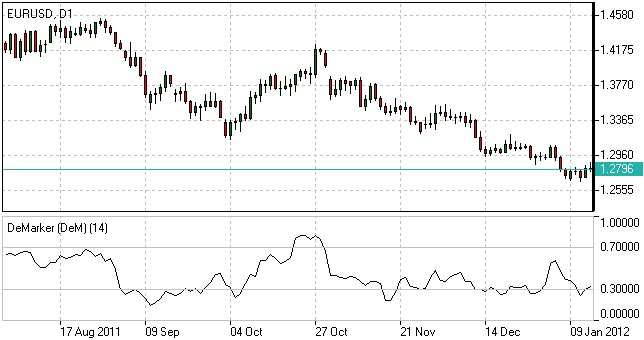# DeMarker Indicator - DeM Indicator

## DeMarker Indicator Definition

The Demarker Indicator is a tool which was introduced by Tom DeMark to help identify emerging buying and selling opportunities. Demarker Indicator demonstrates the price depletion phases which usually correspond with the price highs and bottoms.

Test the Indicator in Action
Once opened Demo you will be supplied with educational materials and online support in your own language

The DeMarker indicator proved to be efficient at identifying trend break-downs as well as spotting intra-day entry and exit points.

## How to Use DeMarker Indicator

DeMarker indicator fluctuates with a range between 0 to 1.

• DeMarker indicator is indicative of lower volatility and a possible price drop when reading 0.7 and higher.
• DeMarker indicator signals a possible price increase when reading below 0.3.Demarker Indicator

## DeMarker Indicator Formula (Calculation)

The DeMarker indicator is the sum of all price increment values recorded during the "i" period divided by the price minima:

```The DeMax(i) is calculated:If high(i) > high(i-1) , then DeMax(i) = high(i)-high(i-1), otherwise DeMax(i) = 0The DeMin(i) is calculated:If low(i) < low(i-1), then DeMin(i) = low(i-1)-low(i), otherwise DeMin(i) = 0The value of the DeMarker is calculated as:DMark(i) = SMA(DeMax, N)/(SMA(DeMax, N)+SMA(DeMin, N))
Where:
SMA - Simple Moving Average;N - the number of periods used in the calculation.

```